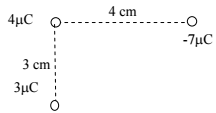Problem: Three point charges +3 μC, +4 μC, and -7 μC are placed along the x and y axis with the values and positions shown. What is the net force, magnitude and direction, acting on the +4 μC charge? (a) 198 N, 37° above +x axis (b) 298 N, 47° above -x axis (c) 342 N, 53° above +x axis (d) 305 N, 53° below +x axis

FREE Expert Solution
80% (310 ratings)
Problem Details

Three point charges +3 μC, +4 μC, and -7 μC are placed along the x and y axis with the values and positions shown. What is the net force, magnitude and direction, acting on the +4 μC charge?

(a) 198 N, 37° above +x axis

(b) 298 N, 47° above -x axis

(c) 342 N, 53° above +x axis

(d) 305 N, 53° below +x axis